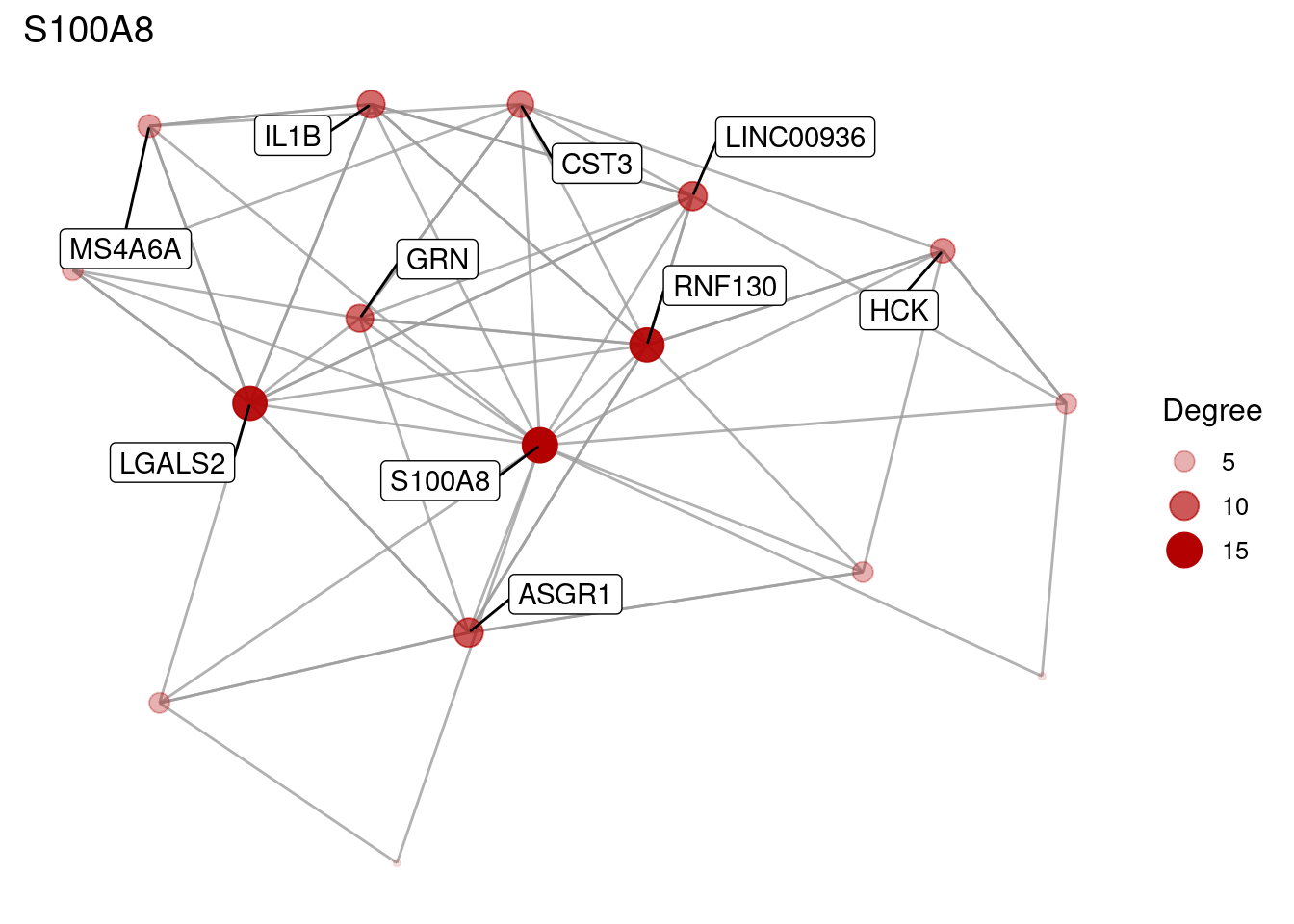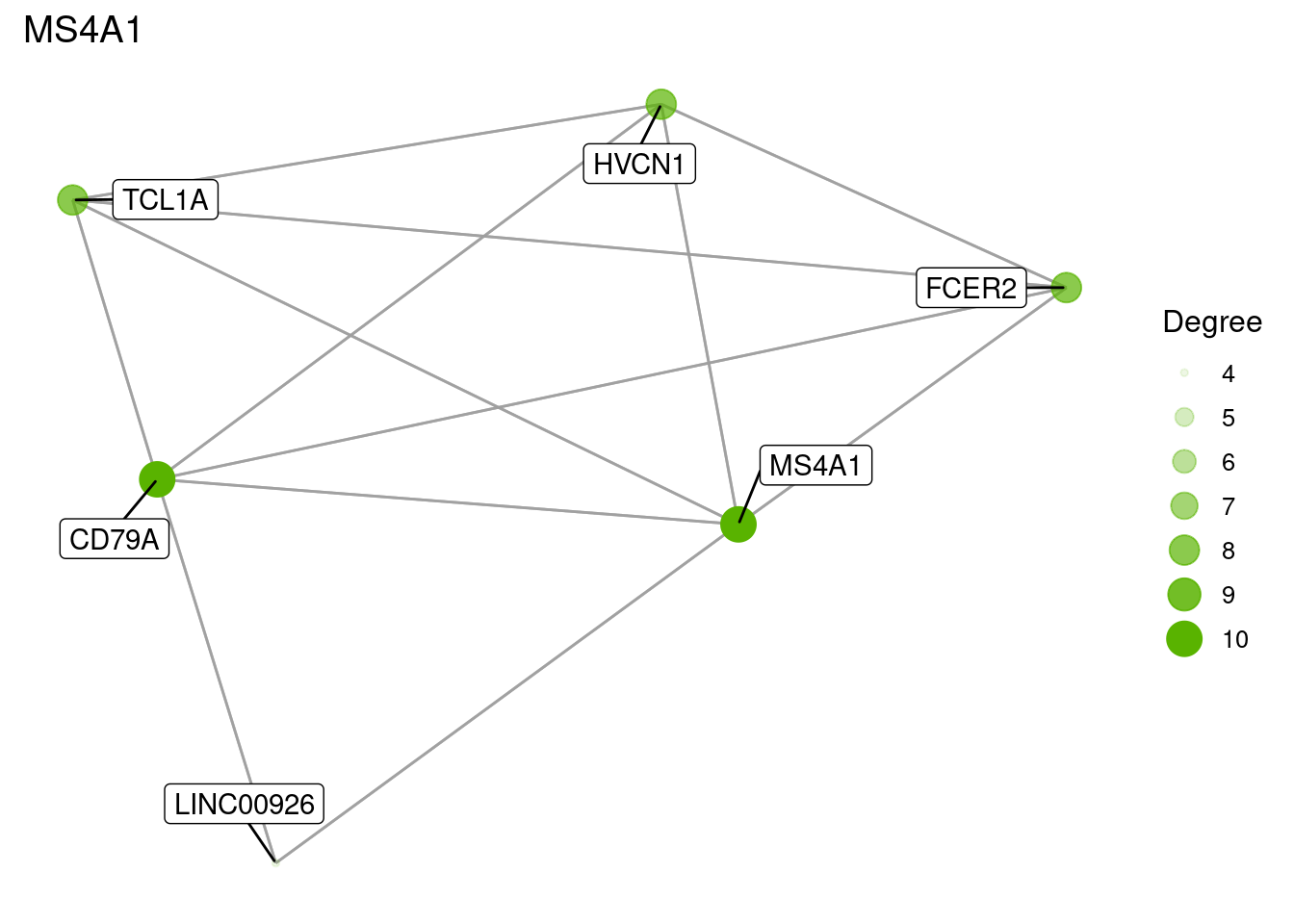## Integration to Seurat

This vignette is targeted at Seurat users that want to use fcoex.

A more in depth explanation of each fcoex step is available in the general vignette, which is based on bioconductor packages.

To integrate a pre-processed Seurat object with fcoex, it is only necessary to obtain the normalized expression matrix and the cluster identities, and then run the pipeline:

``````library(Seurat)
library(fcoex)
library(ggplot2)

data(pbmc_small)

exprs <- data.frame(GetAssayData(pbmc_small))
target <- Idents(pbmc_small)

fc <- new_fcoex(data.frame(exprs),target)``````
``## Created new fcoex object.``
``````fc <- discretize(fc)
fc <- find_cbf_modules(fc,n_genes = 70, verbose = FALSE, is_parallel = FALSE)``````
``## Getting SU scores for each gene``
``## Running FCBF to find module headers``
``````##  "Number of features features =  230"
##  "Number of prospective features =  69"
##  "Number of final features =  9"``````
``## Calculating adjacency matrix``
``## Trimming and getting modules from adjacency matrix``
``## 9 modules were found.``
``fc <- get_nets(fc)``

Let’s take a look at the modules headers:

``mod_names(fc)``
``````##  "S100A8"   "HLA-DPB1" "TYMP"     "HLA-DRB1" "MS4A1"    "LYZ"      "CD7"
##  "IFI6"     "HLA-DQA2"``````

S100A8 is a marker of some granulocytes and MS4A1 is a markers of B cells. Let’s take a look at those two modules.

``mod_names(fc)``
``````##  "S100A8"   "HLA-DPB1" "TYMP"     "HLA-DRB1" "MS4A1"    "LYZ"      "CD7"
##  "IFI6"     "HLA-DQA2"``````
``````network_plots <- show_net(fc)

network_plots[["S100A8"]]````````network_plots[["MS4A1"]]``Once again we can use the Reactome pathways from the package CEMiTool to exemplify how to run an enrichment for human genes. It is likely that some modules will not have any enrichment, leading to messages of the type “no gene can be mapped.”. That is not a problem. If you are not working with human genes, you can just skip this part.

``````gmt_fname <- system.file("extdata", "pathways.gmt", package = "CEMiTool")
fc <- mod_ora(fc, gmt_in)

# In Seurat's sample data, pbmc small, no enrichments are found.
# That is way plot_ora is commented out.

# fc <- plot_ora(fc)``````
``save_plots(name = "fcoex_vignette_Seurat", fc, force = TRUE, directory = "./Plots")``

### Plotting clusters with Seurat

One application of fcoex is to find overlapping populations of cells in the dataset, by employing a module-based view of the gene expression landscape.

Why reclustering? Well, the algorithm behind fcoex considers at the same time inverse and direct correlations. Thus, it is nonsense to obtain a plot of the average expression of the genes in a module, for example.
The reclustering allows us to gather all the signal in the dataset, positive and negative, to see how cells in the dataset behave in relation to that subpart of the transcriptomic space.

Now let’s recluster the cells for some of the fcoex module and visualize it in Seurat .

The cells will be divided on two groups: header positive (HP) and header negative (HN). For details on why, see the next session on anti-correlated genes.

``````library(gridExtra)

fc <- recluster(fc) ``````
``## Detecting clusters for the following modules:``
``##  "S100A8"``
``## S100A8``
``##  "HLA-DPB1"``
``## HLA-DPB1``
``##  "TYMP"``
``## TYMP``
``##  "HLA-DRB1"``
``## HLA-DRB1``
``##  "MS4A1"``
``## MS4A1``
``##  "LYZ"``
``## LYZ``
``##  "CD7"``
``## CD7``
``##  "IFI6"``
``## IFI6``
``##  "HLA-DQA2"``
``## HLA-DQA2``
``````# Running UMAP to obtain layout for cells
pbmc_small <- RunUMAP(pbmc_small, dims = 1:10)``````
``````## Warning: The default method for RunUMAP has changed from calling Python UMAP via reticulate to the R-native UWOT using the cosine metric
## To use Python UMAP via reticulate, set umap.method to 'umap-learn' and metric to 'correlation'
## This message will be shown once per session``````
``## 17:32:42 UMAP embedding parameters a = 0.9922 b = 1.112``
``## 17:32:42 Read 80 rows and found 10 numeric columns``
``## 17:32:42 Using Annoy for neighbor search, n_neighbors = 30``
``## 17:32:42 Building Annoy index with metric = cosine, n_trees = 50``
``## 0%   10   20   30   40   50   60   70   80   90   100%``
``## [----|----|----|----|----|----|----|----|----|----|``
``````## **************************************************|
## 17:32:42 Writing NN index file to temp file /tmp/Rtmp69dsdH/file19cbde29e13866
## 17:32:42 Searching Annoy index using 1 thread, search_k = 3000
## 17:32:42 Annoy recall = 100%
## 17:32:44 Commencing smooth kNN distance calibration using 1 thread with target n_neighbors = 30
## 17:32:44 7 smooth knn distance failures
## 17:32:46 Initializing from normalized Laplacian + noise (using irlba)
## 17:32:46 Commencing optimization for 500 epochs, with 2410 positive edges
## 17:32:48 Optimization finished``````
``````plot1 <- DimPlot(pbmc_small)

pbmc_reclustered <- pbmc_small

# S100A8 is a marker of some monocytes

Idents(pbmc_reclustered) <- idents(fc)[["S100A8"]]
plot2  <- DimPlot(pbmc_reclustered, reduction = 'umap', cols = c("darkgreen", "dodgerblue3")) +
ggtitle("S100A8")

# HLA-DPB1 is a marker of Antigen Presenting Cells
Idents(pbmc_reclustered) <- idents(fc)[["HLA-DPB1"]]
plot3  <- DimPlot(pbmc_reclustered, reduction = 'umap', cols = c("darkgreen", "dodgerblue3")) +
ggtitle("HLA-DPB1")

# MS4A1 is a marker of B cells
Idents(pbmc_reclustered) <- idents(fc)[["MS4A1"]]
plot4  <- DimPlot(pbmc_reclustered, reduction = 'umap', cols = c("darkgreen", "dodgerblue3")) +
ggtitle("MS4A1")

grid.arrange(plot1, plot2, plot3, plot4, nrow=2)``````